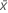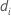# Methods and formulas for Bootstrapping for 2-sample means

Select the method or formula of your choice.

## Mean

A commonly used measure of the center of a batch of numbers. The mean is also called the average. It is the sum of all observations divided by the number of (nonmissing) observations.

### Notation

TermDescription
xiith observation
Nnumber of nonmissing observations

## Standard deviation (StDev)

The sample standard deviation provides a measure of the spread of your data. It is equal to the square root of the sample variance.

### Formula

If the column contains x 1, x 2,..., x N, with mean, then the standard deviation of the sample is:

### Notation

TermDescription
x i i th observationmean of the observations
N number of nonmissing observations

## Variance

The variance measures how spread out the data are about their mean. The variance is equal to the standard deviation squared.

### Notation

TermDescription
xiith observationmean of the observations
Nnumber of nonmissing observations

## Sum

### Notation

TermDescription
xi i th observation

## Minimum

The smallest value in your data set.

## Median

The sample median is in the middle of the data: at least half the observations are less than or equal to it, and at least half are greater than or equal to it.

Suppose you have a column that contains N values. To calculate the median, first order your data values from smallest to largest. If N is odd, the sample median is the value in the middle. If N is even, the sample median is the average of the two middle values.

For example, when N = 5 and you have data x1, x2, x3, x4, and x5, the median = x3.

When N = 6 and you have ordered data x1, x2, x3, x4, x5,and x6:

where x3 and x4 are the third and fourth observations.

## Maximum

The largest value in your data set.

## Average

### Notation

TermDescriptiondifference in means of the ith resamples
B number of resamples
N number of observations for one group in the original sample

## Standard deviation of the bootstrapping distribution

### Notation

TermDescriptionmean of the differences of the resamples
B number of resamplesdifference in means of the ith resample

## Confidence interval

### Formula

Sort the difference in means of the resamples in increasing order. d1 is the lowest number, dB is the highest number.

Lower bound: dl where =Upper bound: du where =###### Note

For a one-sided case (only a lower bound or upper bound), use α instead of α/2.

When l or u are not integers, Minitab does a linear interpolation between the two numbers on either side of l or u. The formula is:

dy + z(dy+1 - dy)

For example, if l = 5.25, the lower bound equals d5 + .25(d6 - d5).

Minitab does not display the confidence interval whenor.

### Notation

TermDescription
α 1- confidence level/100
B number of resamples
dy the yth difference when the data are sorted from least to greatest
y the truncated value of l or u
zl-y or u - y
By using this site you agree to the use of cookies for analytics and personalized content.  Read our policy